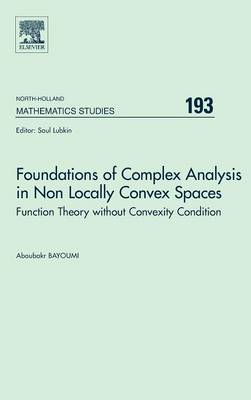•Foundations of Complex Analysis in Non Locally Convex Spaces: Volume 193: Function Theory without Convexity Condition - North-Holland Mathematics Studies (Hardback)

(author)
£145.00
Hardback 304 Pages / Published: 11/11/2003
• Not available

This product is currently unavailable

This product is currently unavailable.

All the existing books in Infinite Dimensional Complex Analysis focus on the problems of locally convex spaces. However, the theory without convexity condition is covered for the first time in this book. This shows that we are really working with a new, important and interesting field. Theory of functions and nonlinear analysis problems are widespread in the mathematical modeling of real world systems in a very broad range of applications. During the past three decades many new results from the author have helped to solve multiextreme problems arising from important situations, non-convex and non linear cases, in function theory. Foundations of Complex Analysis in Non Locally Convex Spaces is a comprehensive book that covers the fundamental theorems in Complex and Functional Analysis and presents much new material. The book includes generalized new forms of: Hahn-Banach Theorem, Multilinear maps, theory of polynomials, Fixed Point Theorems, p-extreme points and applications in Operations Research, Krein-Milman Theorem, Quasi-differential Calculus, Lagrange Mean-Value Theorems, Taylor series, Quasi-holomorphic and Quasi-analytic maps, Quasi-Analytic continuations, Fundamental Theorem of Calculus, Bolzano's Theorem, Mean-Value Theorem for Definite Integral, Bounding and weakly-bounding (limited) sets, Holomorphic Completions, and Levi problem. Each chapter contains illustrative examples to help the student and researcher to enhance his knowledge of theory of functions. The new concept of Quasi-differentiability introduced by the author represents the backbone of the theory of Holomorphy for non-locally convex spaces. In fact it is different but much stronger than the Frechet one. The book is intended not only for Post-Graduate (M.Sc.& Ph.D.) students and researchers in Complex and Functional Analysis, but for all Scientists in various disciplines whom need nonlinear or non-convex analysis and holomorphy methods without convexity conditions to model and solve problems. bull; The book contains new generalized versions of: i) Fundamental Theorem of Calculus, Lagrange Mean-Value Theorem in real and complex cases, Hahn-Banach Theorems, Bolzano Theorem, Krein-Milman Theorem, Mean value Theorem for Definite Integral, and many others. ii) Fixed Point Theorems of Bruower, Schauder and Kakutani's. bull; The book contains some applications in Operations research and non convex analysis as a consequence of the new concept p-Extreme points given by the author. bull; The book contains a complete theory for Taylor Series representations of the different types of holomorphic maps in F-spaces without convexity conditions. bull; The book contains a general new concept of differentiability stronger than the Frechet one. This implies a new Differentiable Calculus called Quasi-differential (or Bayoumi differential) Calculus. It is due to the author's discovery in 1995. bull; The book contains the theory of polynomials and Banach Stienhaus theorem in non convex spaces.

Publisher: Elsevier Science & Technology
ISBN: 9780444500564
Number of pages: 304
Weight: 580 g
Dimensions: 229 x 152 x 18 mm

MEDIA REVIEWS
"...this book is definitely a contribution to the subject..." Richard M. Aron, in: (Mathematical Reviews, 2004)